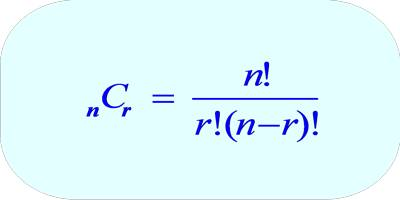In mathematics, a combination is a selection of items from a collection, such that (unlike permutations) the order of selection does not matter. For example, given three fruits, say an apple, an orange, and a pear, there are three combinations of two that can be drawn from this set: an apple and a pear; an apple and an orange; or a pear and an orange.

Combinations are a way to calculate the total outcomes of an event where an order of the outcomes does not matter. To calculate combinations, we will use the formula nCr = n! / r! * (n – r)!, where n represents the total number of items, and r represents the number of items being chosen at a time.

For example, suppose we have a set of three letters: A, B, and C. We might ask how many ways we can select 2 letters from that set. Each possible selection would be an example of a combination. The complete list of possible selections would be: AB, AC, and BC.A combination is an arrangement in which order is NOT important. The notation for combinations is C(n,r) which is the number of combinations of “n” things selected “r” at a time. It is equal to the number of permutations of “n” items taken “r” at a time divided by “r” factorial. This would be P(n,r)/r! in mathematical notation.

Example: If five cards are selected from a group of nine cards, how many combinations of five cards would there be?

The number of possible combinations would be: P(9,5)/5! = (9*8*7*6*5)/(5*4*3*2*1) = 126 possible combinations.

Explanation:

Combination Problem 1: Choose 2 Prizes from a Set of 6 Prizes

You have won first place in a contest and are allowed to choose 2 prizes from a table that has 6 prizes numbered 1 through 6. How many different combinations of 2 prizes could you possibly choose?

In this example, we are taking a subset of 2 prizes (r) from a larger set of 6 prizes (n). Looking at the formula, we must calculate “6 choose 2.”

C (6,2)= 6!/(2! * (6-2)!) = 6!/(2! * 4!) = 15 Possible Prize Combinations

The 15 potential combinations are {1,2}, {1,3}, {1,4}, {1,5}, {1,6}, {2,3}, {2,4}, {2,5}, {2,6}, {3,4}, {3,5}, {3,6}, {4,5}, {4,6}, {5,6}

Combination Problem 2: Choose 3 Students from a Class of 25

A teacher is going to choose 3 students from her class to compete in the spelling bee. She wants to figure out how many unique teams of 3 can be created from her class of 25.

In this example, we are taking a subset of 3 students (r) from a larger set of 25 students (n). Looking at the formula, we must calculate “25 choose 3.”

C (25,3)= 25!/(3! * (25-3)!)= 2,300 Possible Teams.

Information Source: